• /*用1到9的数，组成3个3位数，且三个数比例为1：2：3,求出所有满足条件的数*/ #include #include int noc_1 (int n); //返回n的百位的数字 int noc_2 (int n); //返回n的十位的数字 int noc_3 (int n); //返回n的...
        一个同学在做ACM，给了我一道题，题目就如标题一样。最后写出如下

/*用1到9的数，组成3个3位数，且三个数比例为1：2：3,求出所有满足条件的数*/

#include<stdio.h>
#include<stdbool.h>

int noc_1 (int n);	//返回n的百位的数字
int noc_2 (int n);	//返回n的十位的数字
int noc_3 (int n);	//返回n的个位的数字
bool check (int x,int y,int z);		//检验x y x的每个位上的数字是否相同如果都不相同返回true，否则返回false

int main(void)
{
int a,b,c;	//a b c 为3个三位数的数
int i,j,k;	//i j  k 为a的百 十 个 位的数字
int n_1,n_2,n_3;	//用n_1 n_2 n_3 来轮换a的三个位的数字
for (n_1=1;n_1<4;n_1++)
{
i=n_1;
for (n_2=1;n_2<10;n_2++)
{
if (n_2==n_1)	//如果有相同的数字 舍弃这个系列的组合
continue;
j=n_2;

for (n_3=1;n_3<10;n_3++)
{
if (n_2==n_3||n_1==n_3) //如果有相同的数字 舍弃这个系列的组合
continue;
k=n_3;
a=i*100+j*10+k;
b=a*2;
c=a*3;
if(c>999)	//如果为四位数 舍弃这个组合
continue;
if(check (a,b,c)==true)
printf("%5d%5d%5d\n",a,b,c);
}

}
}
return 0;
}

int noc_1 (int n)
{
return n/100;
}

int noc_2 (int n)
{
n=n/10;
return n%10;
}

int noc_3 (int n)
{
return n%10;
}

bool check (int x,int y,int z)
{
int a;
a=noc_1 (x);
a=noc_2 (x);
a=noc_3 (x);
a=noc_1 (y);
a=noc_2 (y);
a=noc_3 (y);
a=noc_1 (z);
a=noc_2 (z);
a=noc_3 (z);
int i,j;
for (i=0;i<9;i++)
{
if(a[i]==0)		//b c 中可能有位上的数为0 若为0 则返回false
return false;
for(j=0;j<9;j++)
{
if(j==i)
continue;
if(a[i]==a[j])
return false;
}
}
return true;
}编译，运行后如下所示hyp@debian:~$gcc -Wall shu.c hyp@debian:~$ ./a.out
192  384  576
219  438  657
273  546  819
327  654  981
hyp@debian:~$ 这个我觉的应该是有很多的算法的，我没还没看过多少算法的知识，现写成如上。 ------------------------------------------20121127------------------------------- 这个不是我想出来的。确实这个简短多了，也高效多了，我进行了许多无用的思考。事实上，检验可以放到最后，一些判断也可以精简掉。哈哈，长见识了，每个人都有思维的误区，考虑的太草率了。 #include<stdio.h> int main() { int a,b,c,i,j,s; for (a=100;a<333;a++) { b=2*a;c=3*a; s=a%10;s=a%100/10;s=a/100; s=b%10;s=b%100/10;s=b/100; s=c%10;s=c%100/10;s=c/100; for(i=0;i<8;i++) { for(j=i+1;j<9;j++) if(s[i]==s[j]) break; if(j<9) break; } if((i==8)&&(j==9)) printf("%d,%d,%d\n",a,b,c); } return 0; }  展开全文c C • 若一个数能表示某个自然数的平方的形式，则称这个数为完全平方数。 性质： 1. 完全平方数是非负整数。 2. 十进制的完全平方数的末位数只能是0, 1, 4, 5, 6, 9; 十六进制的完全平方数的末位数只能是0, 1, 4,... 定义： 若一个数能表示成某个自然数的平方的形式，则称这个数为完全平方数。性质：1. 完全平方数是非负整数。 2. 十进制的完全平方数的末位数只能是0, 1, 4, 5, 6, 9; 十六进制的完全平方数的末位数只能是0, 1, 4, 9。 3. 除0以外的完全平方数是从1开始的连续的奇数的和，例如: 1 = 1, 4 = 1 + 3, 9 = 1 + 3 + 5, 16 = 1 + 3 + 5 + 7。判断思路：1. 利用定义，即对输入的非负整数开根，得到浮点数，再四舍五入到整数（即最接近的整数），看该整数的平方是否与输入的非负整数相同。 2. 利用性质3，首先判断输入的非负整数是否为0，再判断是否为从1开始的连续的奇数的和（即依次减去1, 3, 5...，直到出现等于0或小于0的结果，等于0则是完全平方数，小于0则不是）优化思路：利用性质2，通过输入的非负整数的末尾数字来过滤不可能是完全平方数的数。在十进制表示下，完全平方数的末位数只能是0, 1, 4, 5, 6, 9，这是比较好理解的（即枚举1到9的平方的末尾数）。同理，在十六进制表示下，我们只需要枚举1到15的平方的末尾数即可，为0，1，4，9，如下所示。0x0 * 0x0 % 0x10 = 0x0 0x1 * 0x1 % 0x10 = 0x1 0x2 * 0x2 % 0x10 = 0x4 0x3 * 0x3 % 0x10 = 0x9 0x4 * 0x4 % 0x10 = 0x0 0x5 * 0x5 % 0x10 = 0x9 0x6 * 0x6 % 0x10 = 0x4 0x7 * 0x7 % 0x10 = 0x1 0x8 * 0x8 % 0x10 = 0x0 0x9 * 0x9 % 0x10 = 0x1 0xa * 0xa % 0x10 = 0x4 0xb * 0xb % 0x10 = 0x9 0xc * 0xc % 0x10 = 0x0 0xd * 0xd % 0x10 = 0x9 0xe * 0xe % 0x10 = 0x4 0xf * 0xf % 0x10 = 0x1 这里有人会问了，为什么取十六进制表示下的末尾数呢？这里我做如下解释： 1. 我们都知道，计算机以二进制来表示数字，位运算速度是最快的，十六进制表示下的末尾数就是二进制下的末4位数，只需要将输入的非负整数与0xF做按位与（&）运算，就可以得到十六进制下的末尾数。因而，得到十六进制下的末尾数比得到十进制下的末尾数要快。 2. 此外，十进制末尾数的过滤比例为1-(5/10)=1/2；十六进制末尾数的过滤比例则为1-(4/16)=3/4；十六进制比十进制能够过滤掉更多的数。 3. 再多说一句，取2^n进制（比如八进制、六十四进制）都是可以的，n越大，过滤掉的数的比例就越高。代码： import math, timeit import matplotlib.pyplot as plt import numpy as np def is_perfect_square_sqrt(n): root = int(math.sqrt(n) + 0.5) return root ** 2 == n # Filter hexadecimal number whose last digit in not in [0, 1, 4, 9] def hex_filter(n): digit = n & 0xf if digit not in [0, 1, 4, 9]: return False else: return True def show_last_digit(): print 'If a number is a perfect square, \ then the last digit of the number in hexadecimal notation must be 0, 1, 4 or 9.' for i in range(16): print "%s * %s %% %s = %s" % (hex(i), hex(i), hex(16), hex((i ** 2) % 16)) # All perfect squares are sums of consecutive odd numbers: # 1 = 1 # 4 = 1 + 3 # 9 = 1 + 3 + 5 # 16 = 1 + 3 + 5 + 7 def is_perfect_square_sub(n): i = 1; while True: if n < 0: return False elif n == 0: return True else: n -= i i += 2 if __name__ == '__main__': # Show the possible last digit of the hexadecimal perfect square number # show_last_digit() # Check if the results of the four methods are the same ''' sqrt = [] sqrt_filter = [] sub = [] sub_filter = [] for i in range(100000): if is_perfect_square_sqrt(i): sqrt.append(i) if is_perfect_square_sub(i): sub.append(i) if hex_filter(i) and is_perfect_square_sqrt(i): sqrt_filter.append(i) if hex_filter(i) and is_perfect_square_sub(i): sub_filter.append(i) print sqrt == sqrt_filter print sub == sub_filter print sqrt == sub print sqrt_filter == sub_filter print sqrt ''' t = [] t.append(timeit.timeit(stmt="for i in range(100000): is_perfect_square_sqrt(i)", setup="from __main__ import is_perfect_square_sqrt", number=1000)) t.append(timeit.timeit(stmt="for i in range(100000): is_perfect_square_sub(i)", setup="from __main__ import is_perfect_square_sub", number=1000)) t.append(timeit.timeit(stmt="for i in range(100000): hex_filter(i) and is_perfect_square_sqrt(i)", setup="from __main__ import is_perfect_square_sqrt, hex_filter", number=1000)) t.append(timeit.timeit(stmt="for i in range(100000): hex_filter(i) and is_perfect_square_sub(i)", setup="from __main__ import is_perfect_square_sub, hex_filter", number=1000)) dic = dict(zip(['sqrt', 'sub', 'sqrt_filter', 'sub_filter'], t)) #print dic for i, key in enumerate(dic): plt.bar(i, dic[key]) # i indicates the i-th bar plt.xticks(np.arange(len(dic)) + 0.4, dic.keys()) plt.yticks(dic.values()) plt.xlabel('Method') plt.ylabel('Running time') plt.show() 运行时间比较： 我们通过循环1000次，每次循环判断从0到99999每个数是否为完全平方数，来比较代码中4种算法的性能。运行时间见下图：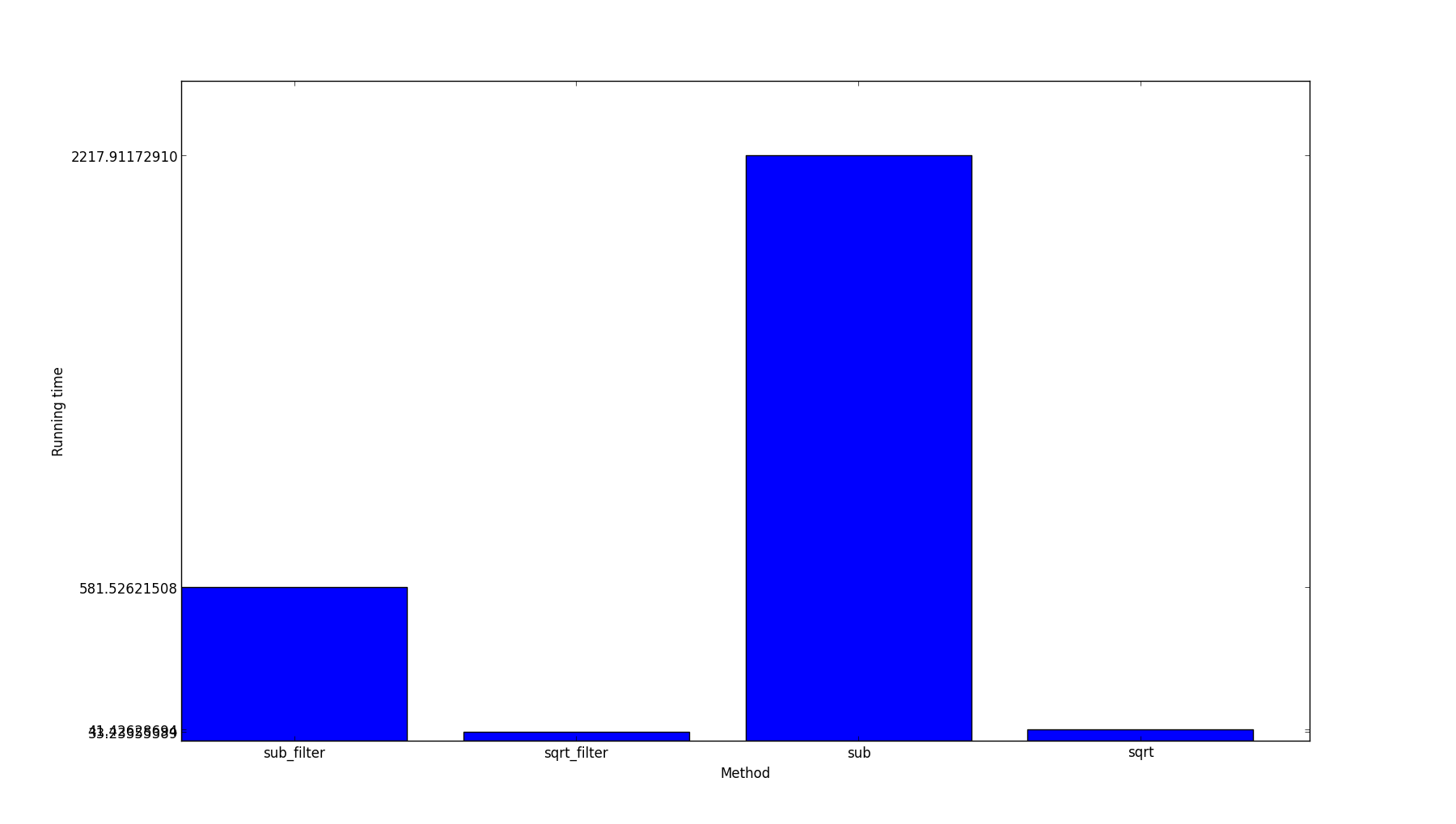由于sqrt_filter和sqrt运行时间较接近，所以y轴的运行时间重叠看不清，分别是33.23355389和41.42628694。 从结果来看，hex_filter优化的时间并没有我想的那么多，可能是因为将hex_filter写作函数，函数调用增加了些额外的时间。  展开全文优化 • 题目要求：将1~9这9个数字分成三组，每组中的三个数一个三位的完全平方数，要求每个数字必须且只能用一次 。 思路如下： 1、先造一个数组num[]用来标记1到9这几个数字是否已经被使用过。比如：num = 0,表示&... 题目要求：将1~9这9个数字分成三组，每组中的三个数排成一个三位的完全平方数，要求每个数字必须且只能用一次 。 思路如下： 1、先造一个数组num[]用来标记1到9这几个数字是否已经被使用过。比如：num = 0,表示"1" 还没被使用过，而 num = 1，则表示"1"前面已经用过了，要重新取数。 每次取数之前都判断一下要取的数对应的num[]标志，这样就解决了 “每个数字必须且只能用一次” 。 2、然后是要造一个三位的数，用三层嵌套循环，比如第一层是遍历百位的数 ，从“1”到“9”一直遍历取数字，后面两层同理，最后造的三位数等于：100x百位的数字+10x十位的数字 +个位的数字； 3、得到一个没有重复数字的三位数后就要判断此数是否为完全平方数。判断的方法很简单，就是判断此数的开方是不是整数，是的话就说明这个数是完全平方数，然后把它打印出来。 重复2、3步的操作，执行程序完后输出的三个数是： 169 256 324 #include <stdio.h> #include <math.h> int judge_perfect_square(int x){ if (sqrt(x) == (int)sqrt(x)) return 1; else return 0; } main(){ int i, x, y, z, ps, num; for(i = 1; i < 10; i++) //Initialize num[] num[i] = 0; for(x = 1; x < 10; x++){ //hundreds if(num[x] == 1) //judge whether the number has already been taken continue; num[x] = 1; //Make a mark for(y = 1; y < 10; y++){ //tens if(num[y] == 1) //Judge whether the number has already been taken continue; num[y] = 1; //Make a mark for(z = 1; z < 10; z++){ //units if(num[z] == 1) //Judge whether the number has already been taken continue; num[z] = 1; //Make a mark ps = 100*x + 10*y + z; //the three-digit number if(judge_perfect_square(ps)) //Judge whether the number is perfect square printf("%d ", ps); else num[z] = 0; //clear the mark if the number is not a perfect square } num[y] = 0; } num[x] = 0; } }   展开全文C语言 数组 • 这实际上就是一个比例控制的问题。 在网上浏览了一些资料,大概有这么几种方式,这里做一归纳。 1.利用counter函数。 一线程就理解用户。 2用户,每用户迭代3次。 一共迭代6次 1.${__counter...
最近项目中遇见了这么一个场景:
100个并发,20个人登录,80个人浏览。

这实际上就是一个比例控制的问题。
在网上浏览了一些资料,大概有这么几种方式,这里做一个归纳。

1.利用counter函数。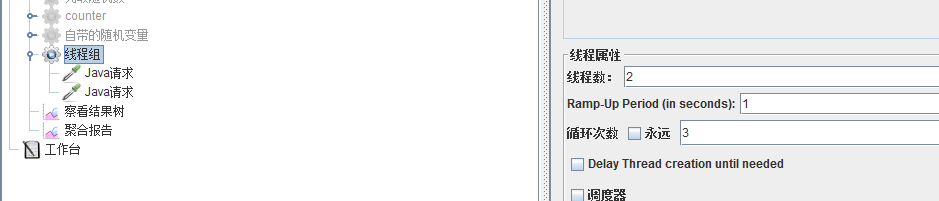一个线程就理解成一个用户。
2个用户,每个用户迭代3次。
一共迭代6次

1.${__counter(true,i)}:只统计该用户的迭代次数，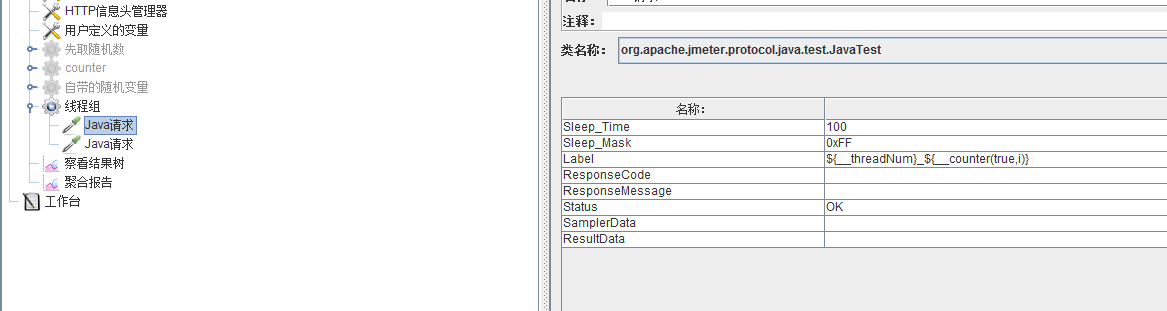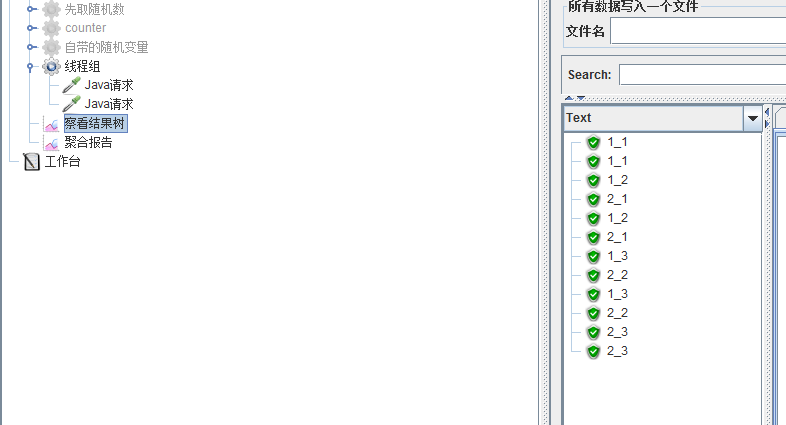2.${__counter(false,i)}:统计所有用户的迭代次数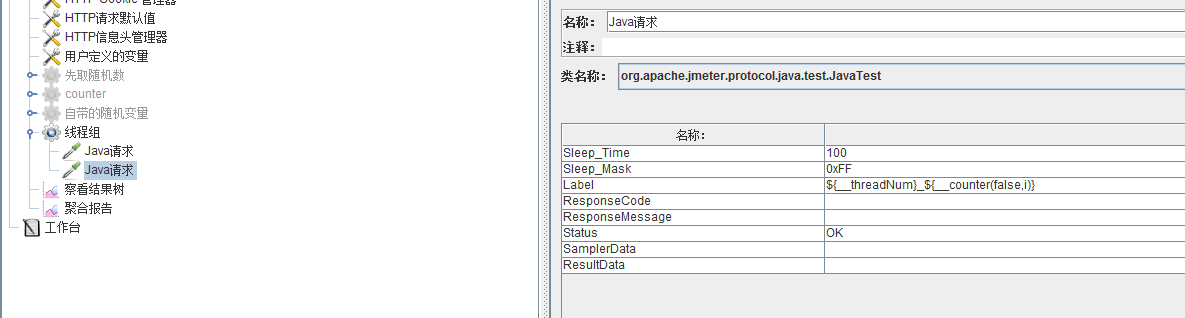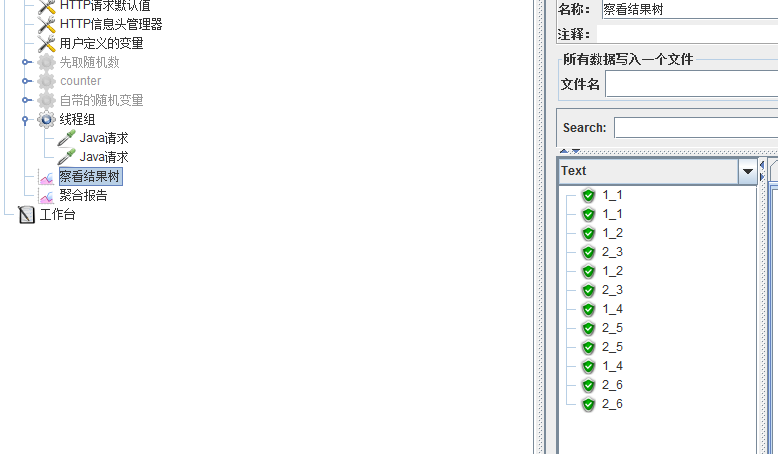如要求比例为2:8。
1,2,3,4,5,6,7,8,9,10。
这10个数中,能被5整除的有5,10;不能被5整除的有余下8个。所以比例为2:8。
写成数学式:
比例为20%，则${__counter(false,)}%5 ==0， 比例为80%，则${__counter(false,)}%5 ！=0
若比例为3:7,
${__counter(false,)}%2 =1,则有1,3,5,7,9${__counter(false,)}%4 =0,则有4,8
${__counter(false,)}%2 =1||${__counter(false,)}%4 =0的集合则为:1,3,4,5,7,8,9
${__counter(false,)}%2 ==0&&${__counter(false,)}%4=2的集合则有2,6,10
则结果为3:7.

说到底是一个数学问题。

这里以2:8为例。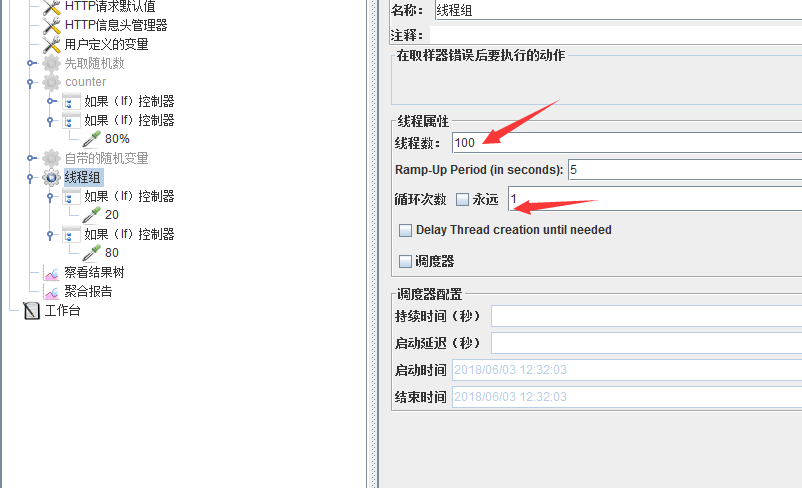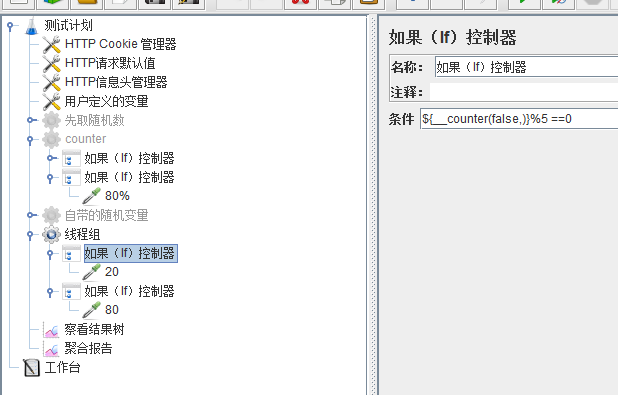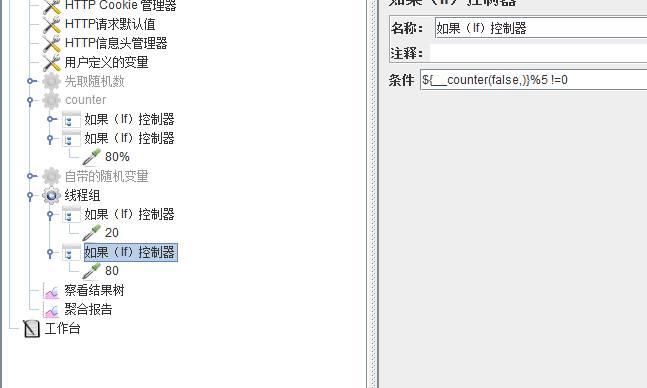${__counter(false,)}%2 =1 这种写法,等价于${__Random(1,10,)}%2==1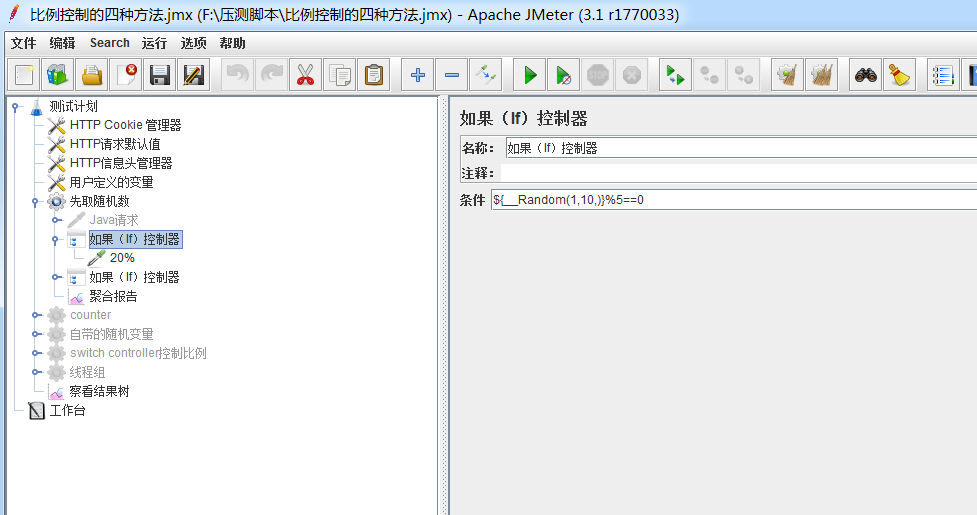方法二:
有些童鞋若觉得通过数学的方法计算比例的方法比较绕,那么可以采用以下的方法。
思路:
取1-10的随机数randNo，
若randNo<=2,执行请求A；randNo>2,执行请求B；

这样A:B=2:8

若randNo<=3,执行请求A；randNo>3,执行请求B；
这样A:B=3:7

这样可以随心所欲的控制比例。

先在前置处理器中生成随机数,用于if控制器中作为条件,进行判断;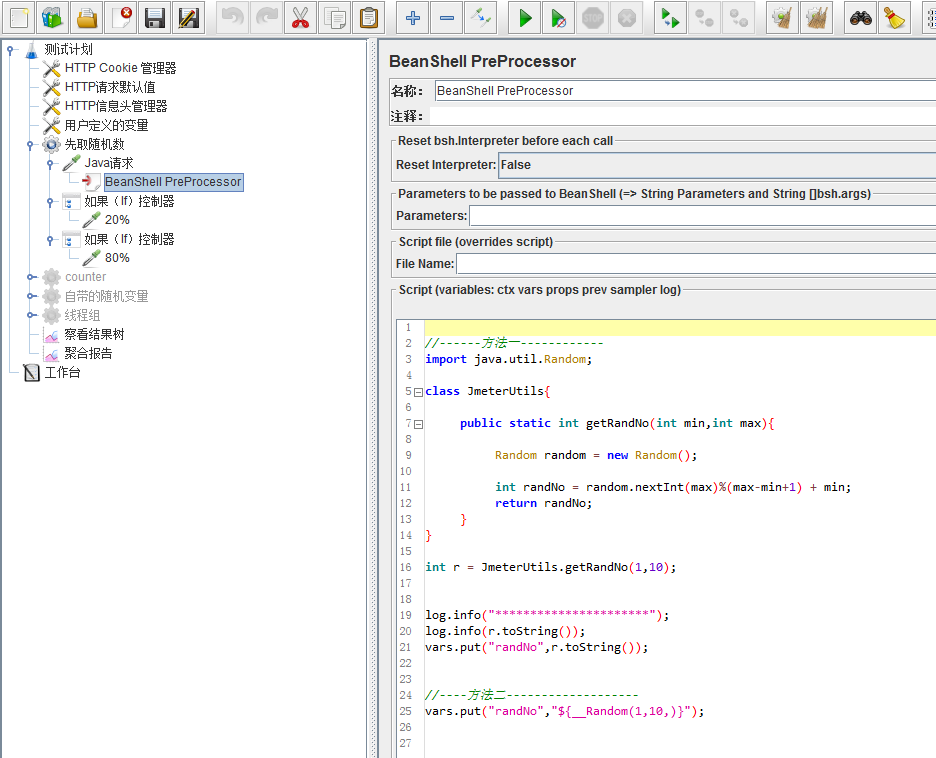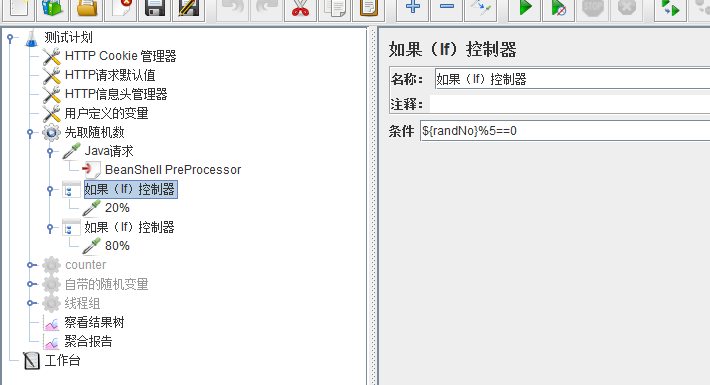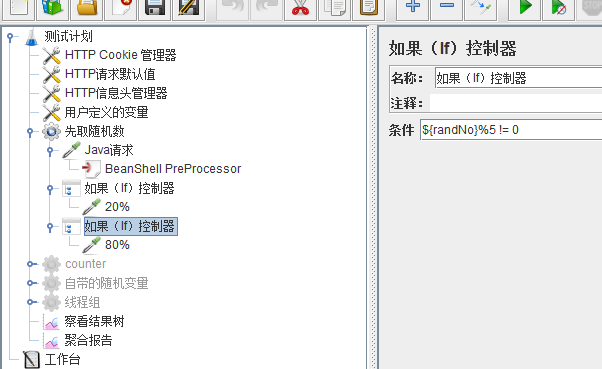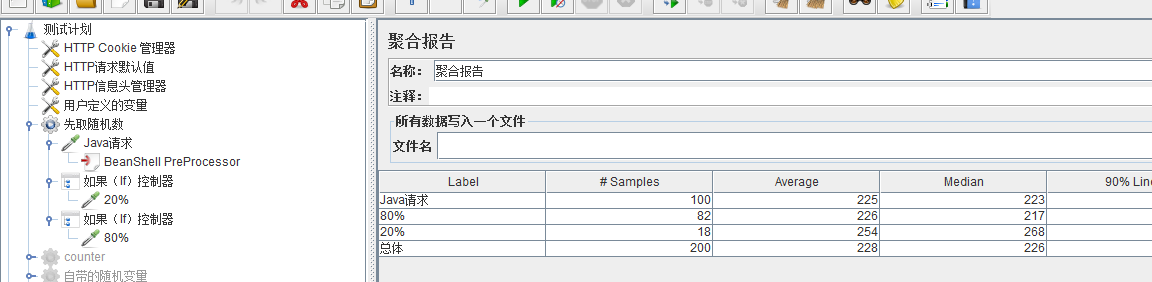但是这种方法每次都要生成随机数,该请求也会对服务器造成一定的压力。
方法三:
思路和方法二一样,只有一点小区别;

是用的配置元件中Random Variable来生成随机数,取代了方法二中在beanshell中生成随机数。
这样就不用增加一个请求,用于生成随机数了,个人认为方法三是优于方法二的。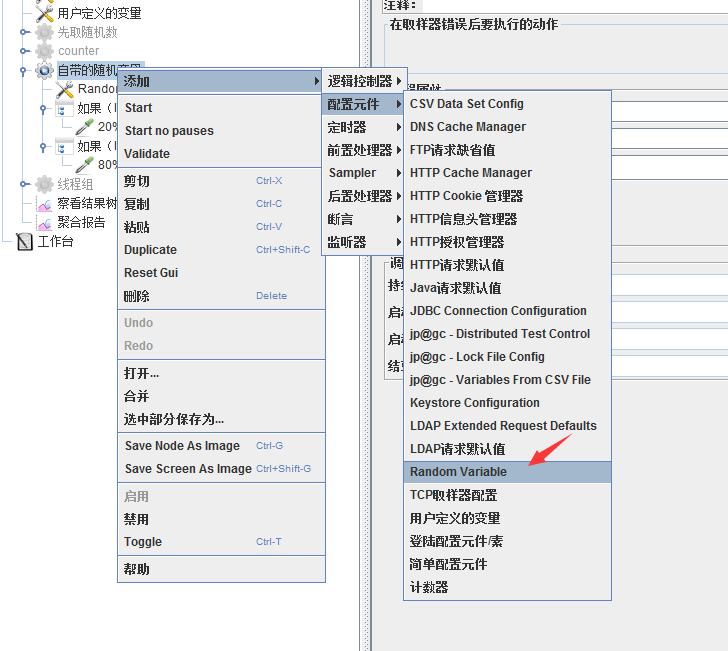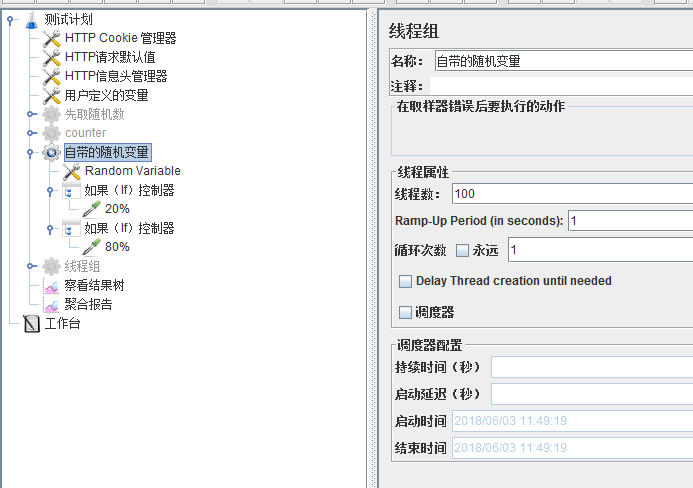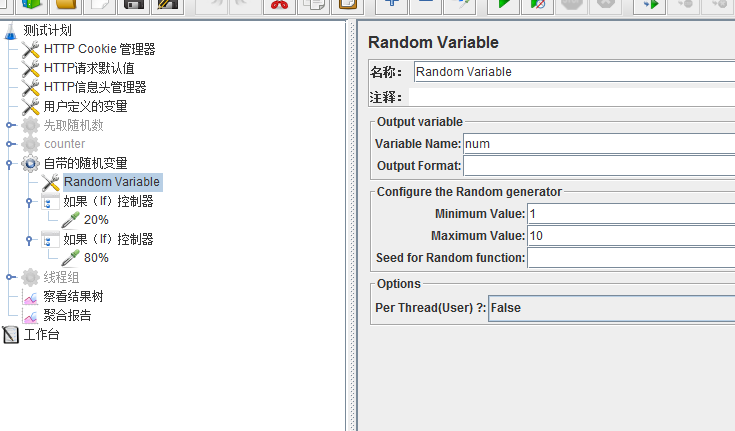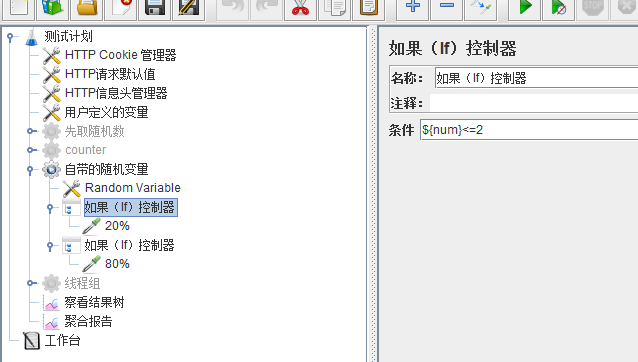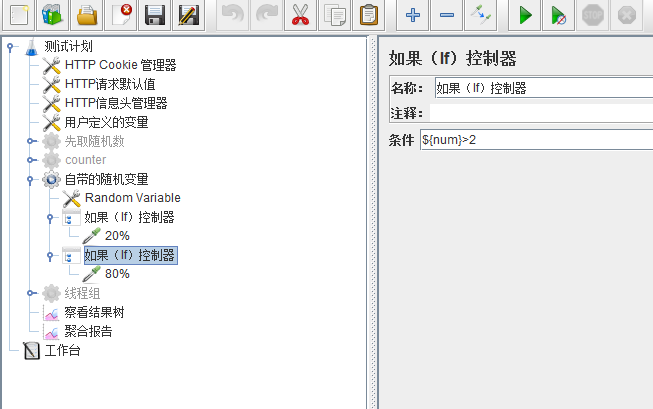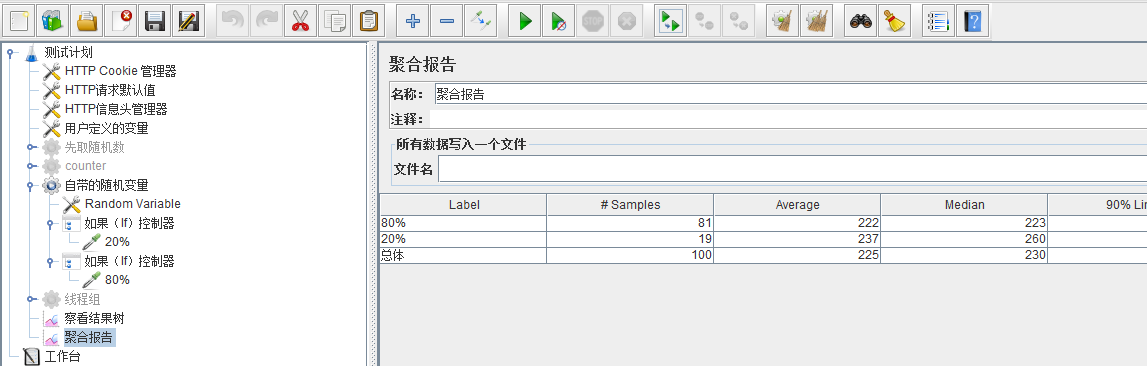方法四:

利用switch controller来实现;

比例2:8,则0,0,1,1,1,1,1,1,1,1

switch的值等于0，执行请求2,switch的值为1执行请求8;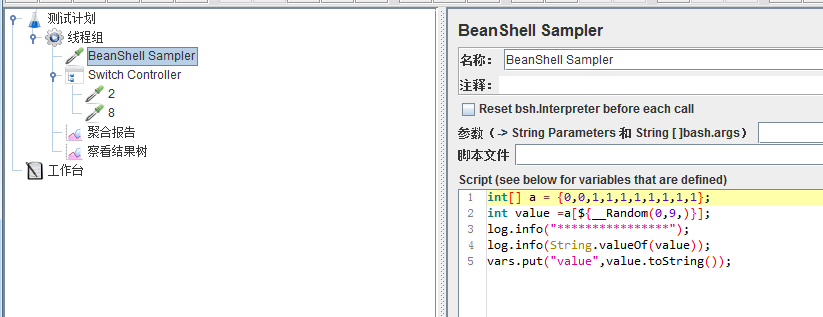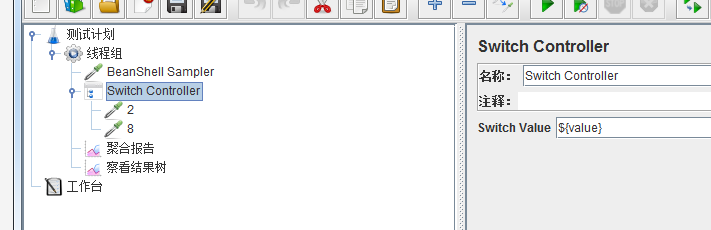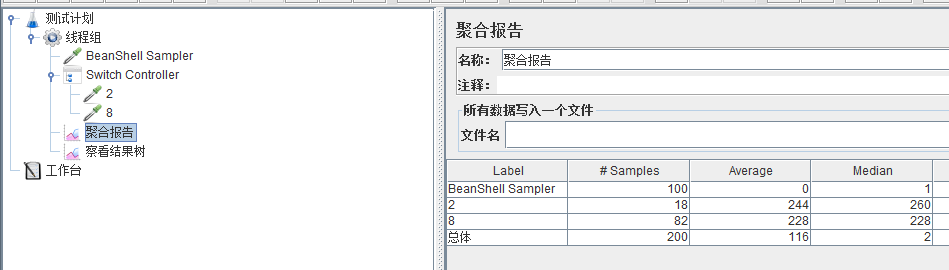这种方法和方法二一样,需要先生成一个值作为参数传入;

优先考虑方法一和方法三,方法二、方法四了解下就可以了,拓展下思路~
练习资料传送门:点击打开链接

展开全文• 原文：http://www.jianshu.com/p/9755da0f4e8f说到Android系统手机，大部分人的印象是用了一段时间就变得有点卡顿，有些程序在运行期间莫名其妙的出现崩溃，打开系统文件夹一看，发现多了很多文件，...相信每使用...
• 一 cpu个数、核数、线程数的关系 cpu个数：是指物理上，也及硬件...线程数：是同一时刻设备能并行执行的程序个数，线程数=cpu个数 * 核数 二 cpu线程数和Java多线程 首先明白几个概念： (1) 单个cpu线程在同...simhash 网页更新
• 内容主要包括分析目标、分析综述、一周运营数据分析、运营数据总体分析块内容 一、 确定分析目标 分析目标主要包括以下三方面： ● 分析目的； ● 分析范围； ● 分析时间。 如下图所示，分析目标除了主要...
• 为其所在团队开发一款调查问卷形式的小程序，其中有一个主要功能是需要用户上传所处位置东南西北四个方向的照片，然后在服务端对这四张图片进行解析，提取出图片中人眼所见绿色所占整张图片的比例。 打个比方，如下...
• 计算颜色直方图需要将颜色空间划分若干小的颜色区间，每小区间成为直方图的一bin。这过程称为颜色量化（color quantization）。然后，通过计算颜色落在每小区间内的像素数量可以得到颜色直方图。颜色...
• 点赞再看，养成习惯，微信搜索【三太子敖丙】关注这互联网苟且偷生的工具人。 本文 GitHub https://github.com/JavaFamily 已收录，有一线大厂面试完整考点、资料以及我的系列文章。 前言 前段时间敖丙不是在复习...后端 java
•优化 前端 web应用开发
• 作业要求： 1、输入：有一张写着数字的A4纸的图片（如下） ...4、用Adaboost或SVM训练一手写数字分类器 5、识别并输出：连串数字，如“13924579693”与“02087836761”等 实现环境： Windows10 + VS2015 + cimg库计算机视觉 手写数字识别 字符分割
• 需要把旧的推荐服务逐步切换到新的推荐服务上，需要灰度切换，流量比例和灰度策略可以控制。   二. 方案 当前数据请求流程是：外部请求—&gt;易车nginx ---&gt;后端服务 ； 经过跟运维沟通发现，目前...openresty
• 2013年房地产投资占GDP比例高达16%，而事实上从1960年来但凡房地产投资占GDP比例高于6%的国家，房地产泡沫都出现了破灭。而事实上，当年日本房地产泡沫破灭时，房地产投资占GDP比重也不过是9%，而美国次贷危机爆发时...
• 华电北风吹 天津大学认知计算与应用重点实验室 ...所以只需要让第一值为初始最大值或者初始最小值，用所有的值与这值比较，更新这值即可。def minimum(a): minNum=a for i in range(1,len(a)):顺序统计量 快速排序
• ## BigDecimal整除判断

千次阅读 2019-08-27 14:59:55
展示包装数量时，需要进行包装单位换算，一级包装单位换二级单位，当能换二级单位时（大），则显示二级单位，否则显示一级单位（小）。 如果库存数量是120盒牛奶，直接显示10箱； 如果库存数量是100盒牛奶，直接...
• 部分:数字推理题典！！16 (数字的整除特性)62 继续题典65 本题典说明如下：本题典的所有题都适用！ 1）题目部分用黑体字 2）解答部分用红体字 3）先给出的是题目，解答在题目后。 4）如果一题目有多种...数字推理题
• 但是操作系统对一进程内的线程还是有限制的，不能无限生成，经验值在3000~5000左右。 java -Xmx3550m -Xms3550m -Xss128k  -XX:NewRatio=4 -XX:SurvivorRatio=4 -XX:MaxPermSize=16m -XX:...java
• 怎么判断呢? 欠拟合 机器学习中一重要的话题便是模型的泛化能力，泛化能力强的模型才是好模型，对于训练好的模型，若在训练集表现差，在测试集表现同样会很差，这可能是欠拟合导致, 这是泛化能力太强, 训练集上和...
•  这看似很神奇的过程，其实很简单。强光是电影放映机的大灯泡发出来的，那灯泡通常有1万瓦，非常亮哈。然后强光把电影胶片上的图像打到银幕上，就这么简单。如果没有胶片，银幕上就是一片白色。看过幻灯片吧，跟...
• 核心层：数字经济发展的底层建筑核心层的范畴及其经济含义从宏观视角看，核心层是支撑数字经济发展的底层建筑。这基座主要包括（1）体、 （2）信息技术（IT）、（3）通信技术、（4）智能硬件...lamp makefile lighttpd
• 结果一K-邻近实现手写数字识别的代码就让我改了三天。虽然网上这方面的代码是很多，但是我运行了好几，结果都不是很理想。一次偶然的念想——为什么我不把这些代码的优点结合在一起呢，于是说做就做，年轻人嘛...knn算法
• 、无标度网络 原文：Chapter 4 Scale-free networks 译者：飞龙 协议：CC BY-NC-SA 4.0 自豪地采用谷歌翻译 在本章中，我们将处理来自在线社交网络的数据，并使用 WS 图对其进行建模。WS 模型像数据一样...
• 每个.mat都是一个 若干行x784 的二维矩阵，其行数代表数据条数，列数的784是28的平方，也就是一张数字手写体图像的像素个数(每张数字手写体是 28x28 的灰度图)。    说道灰度图，那就还有一个问题值得考虑：需...数字手写体识别 图像处理 朴素贝叶斯分类器 MATLAB 机器学习
• 主要体现在以下四个方面。 （一）颠覆全球个人支付方式 在数字化浪潮来临之前，我国个人支付主要通过纸币、储蓄卡、信用卡来完成。2002年，在合并了全国银行卡信息交换总中心和18家城市（区域）银行卡网络服务分中心...
• 1.概述图像识别的一核心问题是图像的特征提取，简单描述即为用一组简单的数据(数据描述量)来描述整个图像，这组数据月简单越有代表性越好。良好的特征不受光线、噪点、几何形变的干扰，图像识别技术的发展中，不断...图像的矩 图像中心矩 图像质心 图像轮廓面积
• 但是大量重要的ILP和INLP问题，并不存在多项式时间的解法，因此，启发式算法可以这样定义：一基于直观或经验构造的算法，在可接受的花费（指计算时间和空间）下给出待解决组合优化问题每一实例的一可行解，该...
• 其实这次的代码绘制的只是一简单的三角形。只是这样，却写了这么长的代码，所以才说3D开发是比较难的。 但是，个人认为，即使这样，和DirectX相比较的话，已经相当简单，简练了。 单从开发环境上来说，不需要特别...WebGL
• 下面从四个角度进一步去判断业务的可行性。 第一，数据层面。 这应该是区块链技术在这个场景下最大的价值所在。通过分布式总账的建立，实现数据的分布式记录，而不是存储在某一个中心服务器上，并且数据按照时间先后......